# sklearn.datasets.make_blobs¶

sklearn.datasets.make_blobs(n_samples=100, n_features=2, *, centers=None, cluster_std=1.0, center_box=(-10.0, 10.0), shuffle=True, random_state=None, return_centers=False)[source]

Generate isotropic Gaussian blobs for clustering.

Read more in the User Guide.

Parameters:
n_samplesint or array-like, default=100

If int, it is the total number of points equally divided among clusters. If array-like, each element of the sequence indicates the number of samples per cluster.

Changed in version v0.20: one can now pass an array-like to the n_samples parameter

n_featuresint, default=2

The number of features for each sample.

centersint or array-like of shape (n_centers, n_features), default=None

The number of centers to generate, or the fixed center locations. If n_samples is an int and centers is None, 3 centers are generated. If n_samples is array-like, centers must be either None or an array of length equal to the length of n_samples.

cluster_stdfloat or array-like of float, default=1.0

The standard deviation of the clusters.

center_boxtuple of float (min, max), default=(-10.0, 10.0)

The bounding box for each cluster center when centers are generated at random.

shufflebool, default=True

Shuffle the samples.

random_stateint, RandomState instance or None, default=None

Determines random number generation for dataset creation. Pass an int for reproducible output across multiple function calls. See Glossary.

return_centersbool, default=False

If True, then return the centers of each cluster.

New in version 0.23.

Returns:
Xndarray of shape (n_samples, n_features)

The generated samples.

yndarray of shape (n_samples,)

The integer labels for cluster membership of each sample.

centersndarray of shape (n_centers, n_features)

The centers of each cluster. Only returned if return_centers=True.

make_classification

A more intricate variant.

Examples

>>> from sklearn.datasets import make_blobs
>>> X, y = make_blobs(n_samples=10, centers=3, n_features=2,
...                   random_state=0)
>>> print(X.shape)
(10, 2)
>>> y
array([0, 0, 1, 0, 2, 2, 2, 1, 1, 0])
>>> X, y = make_blobs(n_samples=[3, 3, 4], centers=None, n_features=2,
...                   random_state=0)
>>> print(X.shape)
(10, 2)
>>> y
array([0, 1, 2, 0, 2, 2, 2, 1, 1, 0])


## Examples using sklearn.datasets.make_blobs¶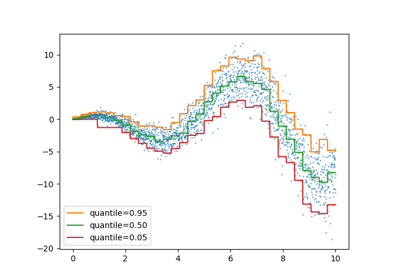Release Highlights for scikit-learn 1.1

Release Highlights for scikit-learn 1.1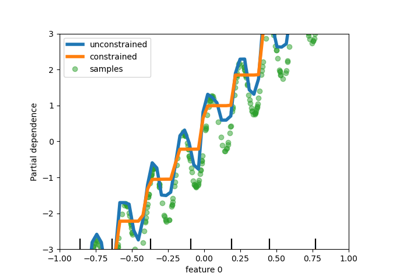Release Highlights for scikit-learn 0.23

Release Highlights for scikit-learn 0.23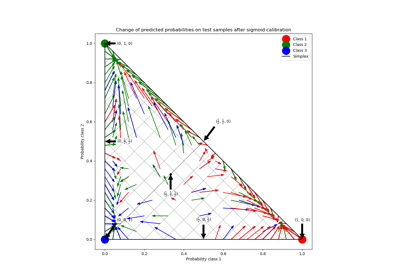Probability Calibration for 3-class classification

Probability Calibration for 3-class classification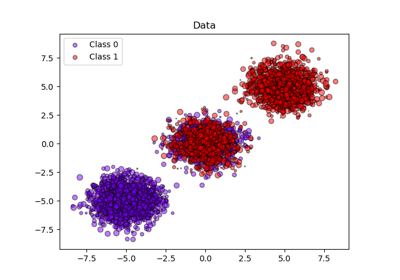Probability calibration of classifiers

Probability calibration of classifiers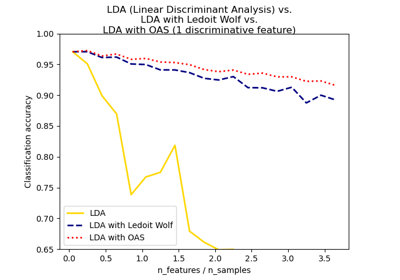Normal, Ledoit-Wolf and OAS Linear Discriminant Analysis for classification

Normal, Ledoit-Wolf and OAS Linear Discriminant Analysis for classification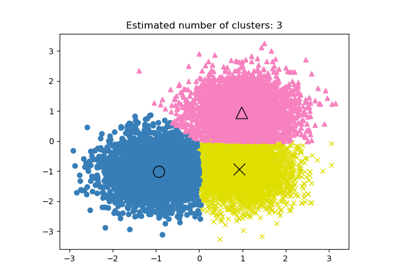A demo of the mean-shift clustering algorithm

A demo of the mean-shift clustering algorithm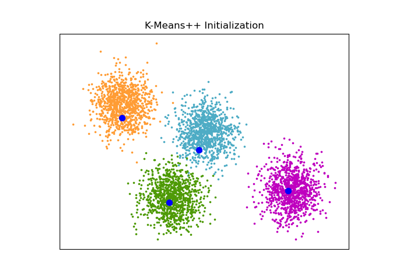An example of K-Means++ initialization

An example of K-Means++ initialization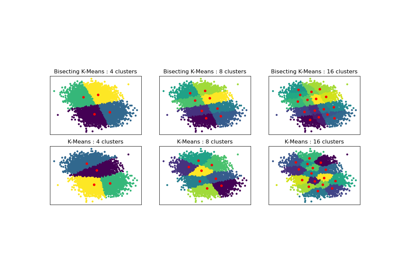Bisecting K-Means and Regular K-Means Performance Comparison

Bisecting K-Means and Regular K-Means Performance Comparison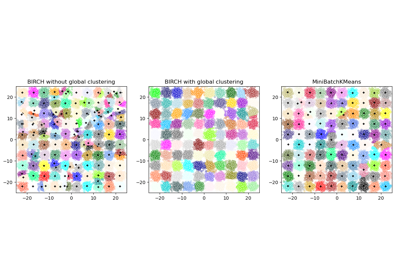Compare BIRCH and MiniBatchKMeans

Compare BIRCH and MiniBatchKMeans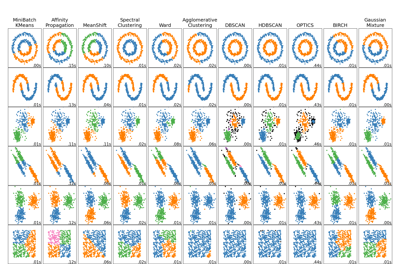Comparing different clustering algorithms on toy datasets

Comparing different clustering algorithms on toy datasets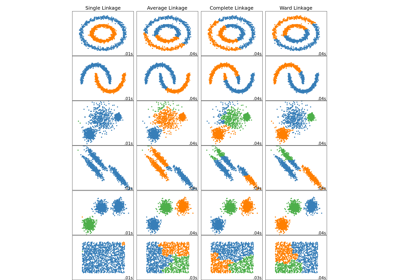Comparing different hierarchical linkage methods on toy datasets

Comparing different hierarchical linkage methods on toy datasets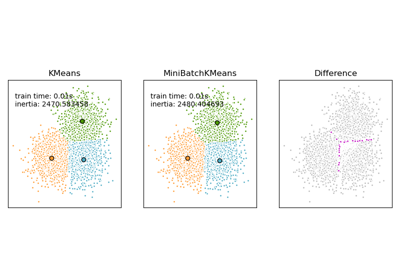Comparison of the K-Means and MiniBatchKMeans clustering algorithms

Comparison of the K-Means and MiniBatchKMeans clustering algorithms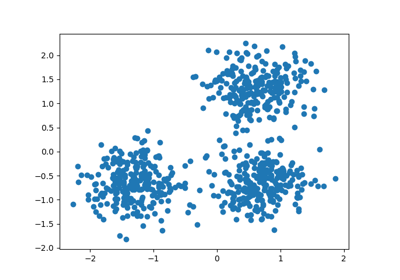Demo of DBSCAN clustering algorithm

Demo of DBSCAN clustering algorithm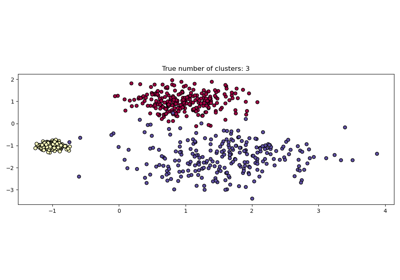Demo of HDBSCAN clustering algorithm

Demo of HDBSCAN clustering algorithm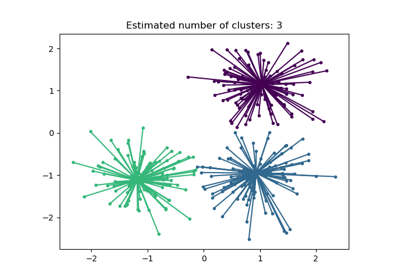Demo of affinity propagation clustering algorithm

Demo of affinity propagation clustering algorithmDemonstration of k-means assumptions

Demonstration of k-means assumptions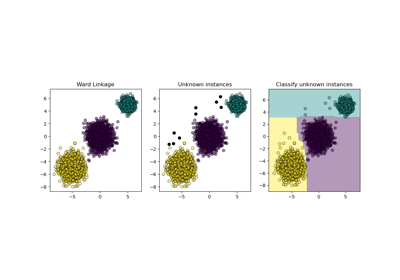Inductive Clustering

Inductive Clustering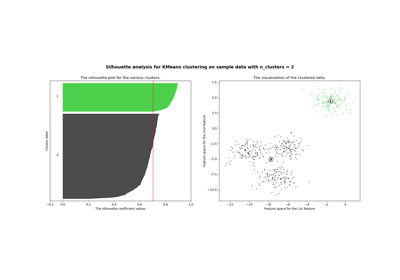Selecting the number of clusters with silhouette analysis on KMeans clustering

Selecting the number of clusters with silhouette analysis on KMeans clusteringPlot randomly generated classification dataset

Plot randomly generated classification datasetGMM Initialization Methods

GMM Initialization Methods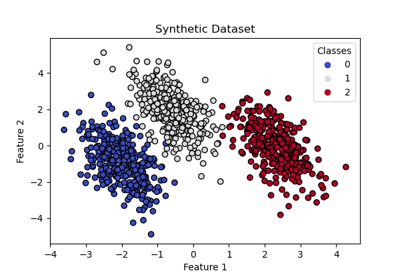Plot multinomial and One-vs-Rest Logistic Regression

Plot multinomial and One-vs-Rest Logistic Regression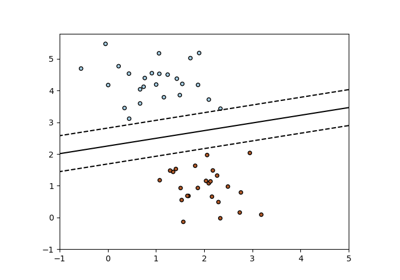SGD: Maximum margin separating hyperplane

SGD: Maximum margin separating hyperplane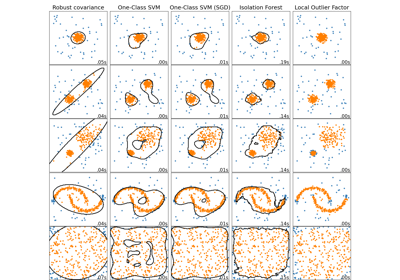Comparing anomaly detection algorithms for outlier detection on toy datasets

Comparing anomaly detection algorithms for outlier detection on toy datasets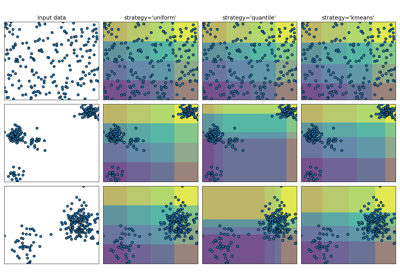Demonstrating the different strategies of KBinsDiscretizer

Demonstrating the different strategies of KBinsDiscretizer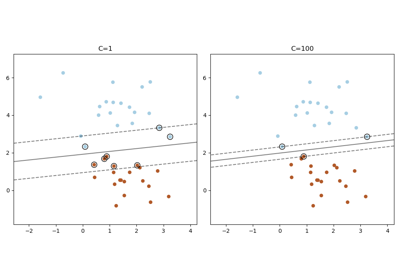Plot the support vectors in LinearSVC

Plot the support vectors in LinearSVC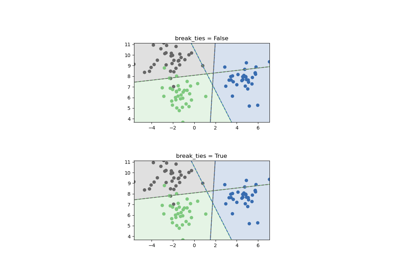SVM Tie Breaking Example

SVM Tie Breaking Example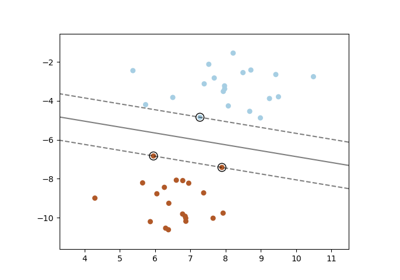SVM: Maximum margin separating hyperplane

SVM: Maximum margin separating hyperplane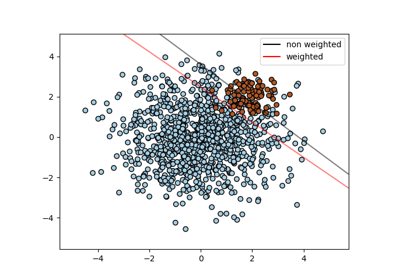SVM: Separating hyperplane for unbalanced classes

SVM: Separating hyperplane for unbalanced classes